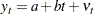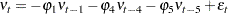The AUTOREG Procedure
 Stepwise Autoregression

Once you determine that autocorrelation correction is needed, you must select the order of the autoregressive error model to use. One way to select the order of the autoregressive error model is stepwise autoregression. The stepwise autoregression method initially fits a high-order model with many autoregressive lags and then sequentially removes autoregressive parameters until all remaining autoregressive parameters have significant t tests.

To use stepwise autoregression, specify the BACKSTEP option, and specify a large order with the NLAG= option. The following statements show the stepwise feature, using an initial order of 5:

```/*-- stepwise autoregression --*/
proc autoreg data=a;
model y = time / method=ml nlag=5 backstep;
run;
```

The results are shown in Figure 8.9.

Figure 8.9 Stepwise Autoregression
 Forecasting Autocorrelated Time Series

The AUTOREG Procedure

Dependent Variable y

Ordinary Least Squares Estimates
SSE 214.953429 DFE 34
MSE 6.32216 Root MSE 2.51439
SBC 173.659101 AIC 170.492063
MAE 2.01903356 AICC 170.855699
MAPE 12.5270666 HQC 171.597444
Durbin-Watson 0.4752 Regress R-Square 0.8200
Total R-Square 0.8200

Parameter Estimates
Variable DF Estimate Standard Error t Value Approx
Pr > |t|
Intercept 1 8.2308 0.8559 9.62 <.0001
time 1 0.5021 0.0403 12.45 <.0001

Estimates of Autocorrelations
Lag Covariance Correlation -1 9 8 7 6 5 4 3 2 1 0 1 2 3 4 5 6 7 8 9 1
0 5.9709 1.000000 |                    |********************|
1 4.5169 0.756485 |                    |***************     |
2 2.0241 0.338995 |                    |*******             |
3 -0.4402 -0.073725 |                   *|                    |
4 -2.1175 -0.354632 |             *******|                    |
5 -2.8534 -0.477887 |          **********|                    |

Backward Elimination of Autoregressive
Terms
Lag Estimate t Value Pr > |t|
4 -0.052908 -0.20 0.8442
3 0.115986 0.57 0.5698
5 0.131734 1.21 0.2340

The estimates of the autocorrelations are shown for 5 lags. The backward elimination of autoregressive terms report shows that the autoregressive parameters at lags 3, 4, and 5 were insignificant and eliminated, resulting in the second-order model shown previously in Figure 8.4. By default, retained autoregressive parameters must be significant at the 0.05 level, but you can control this with the SLSTAY= option. The remainder of the output from this example is the same as that in Figure 8.3 and Figure 8.4, and it is not repeated here.

The stepwise autoregressive process is performed using the Yule-Walker method. The maximum likelihood estimates are produced after the order of the model is determined from the significance tests of the preliminary Yule-Walker estimates.

When using stepwise autoregression, it is a good idea to specify an NLAG= option value larger than the order of any potential seasonality, since seasonality produces autocorrelation at the seasonal lag. For example, for monthly data use NLAG=13, and for quarterly data use NLAG=5.

### Subset and Factored Models

In the previous example, the BACKSTEP option dropped lags 3, 4, and 5, leaving a second-order model. However, in other cases a parameter at a longer lag may be kept while some smaller lags are dropped. For example, the stepwise autoregression method might drop lags 2, 3, and 5 but keep lags 1 and 4. This is called a subset model, since the number of estimated autoregressive parameters is lower than the order of the model.

Subset models are common for seasonal data and often correspond to factored autoregressive models. A factored model is the product of simpler autoregressive models. For example, the best model for seasonal monthly data may be the combination of a first-order model for recent effects with a 12th-order subset model for the seasonality, with a single parameter at lag 12. This results in a 13th-order subset model with nonzero parameters at lags 1, 12, and 13. See Chapter 7, The ARIMA Procedure, for further discussion of subset and factored autoregressive models.

You can specify subset models with the NLAG= option. List the lags to include in the autoregressive model within parentheses. The following statements show an example of specifying the subset model resulting from the combination of a first-order process for recent effects with a fourth-order seasonal process:

```/*-- specifying the lags --*/
proc autoreg data=a;
model y = time / nlag=(1 4 5);
run;
```

The MODEL statement specifies the following fifth-order autoregressive error model:Previous Page | Next Page | Top of Page